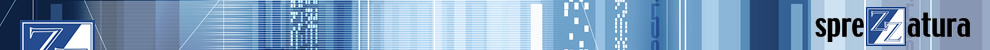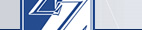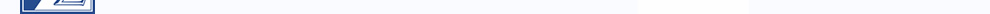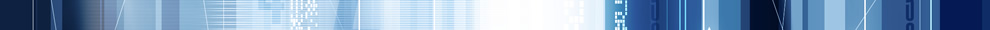In order for this site to work correctly, and for us to improve the site, we need to store a small file (called a cookie) on your computer.# RevMedia

1. Volume 1
2. Volume 2
3. Volume 3
4. Volume 4

### QTIPS - FOR/NEXT variables

Tony McDowell writes - "The documentation (page N4.73) states that "start", "end" and "step" must be integers. A little experimentation might suggest that in fact any decimal fractions can be used for these variables. Don't be fooled. If STEP is a decimal fraction then anomalous results can occur. Specifically, it seems that the "even-ness" of the bound and step variables is critical. My tests show that if step and the lower and upper bounds are "even" decimal fractions then the FOR/NEXT loop will incorrectly terminate when step is equal to "end" rather than when it exceeds "end" (or underflows if step is negative)."

0001       * This one is bad - it won't hit -2.0
0002       A = 2 ; B = -2
0003       For I = A To B Step -0.2 ; Call Msg("I = " : I) ; Next
0004
0005       * This one is OK
0006       A = 1 ; B = -1
0007       For I = A To B Step -0.2 ; Call Msg("I = " : I) ; Next
0008
0009       * This one OK
0010       A = 2 ; B = 4
0011       For I = A To B Step -0.5 ; Call Msg("I = " : I) ; Next
0012
0013       * This one is bad - it won't hit 4.0
0014       A = 2 ; B = 4
0015       For I = A To B Step -0.4 ; Call Msg("I = " : I) ; Next

* NB Version 2.12 MSG used above!

(Volume 4, Issue 2, Page 5)Making Databases Happen Registered Address: 12A Marlborough Place, Brighton, BN1 1WN UK +44 (0) 20 8912 1010 USA +1 215 939 3400